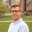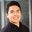Question-and-Answer Resource for the Building Energy Modeling Community
Get s tarted with the Help page

# Is there a way to extract thermal mass from an energyplus model?I am comparing different building designs and would like to understand the role of thermal mass for their energy performance better. Is there a way to extract the total available thermal mass in a building model or in a zone, e.g. in J/m2K?

Update

After receiving two fantastic answers, I realised that I should have phrased my question a bit more specifically. Technically, heat capacity will not only depend on a material's thickness, density, and heat capacity, but also on dynamic effects, such as amplitude, etc. One rule of thumb is that only the first few centimetres in a surface have relevant thermal inertia. So I was wondering if any kind of measure could be extracted form e+.

Not sure if this update warrants for a new question...

edit retag close merge delete

Sort by » oldest newest most votedTo do it programmatically, you could either use OpenStudio and Ruby or EnergyPlus and Python.

OpenStudio and Ruby

Import the IDF to OpenStudio and as @Luis Lara suggested run a Ruby script (or formal OpenStudio Measure) on the OpenStudio model.

EnergyPlus and Python

Here's a lightly tested Python script (using eppy). Depending on your model, you may want to add filters (e.g. Construction:InternalSource, Material:NoMass, etc.).

import os
from eppy.modeleditor import IDF

idd_dir = r'C:\EnergyPlusV9-1-0'
idd_file = r'Energy+.idd'
idd_path = os.path.join(idd_dir, idd_file)

idf_dir = r'C:\WORK\18151 DEN Level 4\18151-Baseline\run'
idf_file = r'in.idf'
idf_path = os.path.join(idf_dir, idf_file)

IDF.setiddname(idd_path)

idf = IDF(idf_path)

total_heat_capacity = 0

surfaces = idf.idfobjects['BuildingSurface:Detailed'.upper()]

# loop through the surfaces to calculate heat capacity
for surface in surfaces:

construction_heat_capacity = 0

construction = idf.getobject('CONSTRUCTION', surface.Construction_Name)

# get the field names, which in eppy is a list of
# ['key', 'Name', 'Outside_Layer', 'Layer_2', ... 'Layer_10']
construction_field_names = construction.fieldnames

# loop through the construction's field names (layers),
# skipping first and second (key and Name)
for field_name in construction_field_names[2:]:

# get the material object from the layer
attribute = field_name
material_name = getattr(construction, attribute)
material = idf.getobject('MATERIAL', material_name)

# calculate the material's heat capacity and add it to the construction's
if material is not None:
thickness = material.Thickness
density = material.Density
specific_heat = material.Specific_Heat
material_heat_capacity = thickness * density * specific_heat
construction_heat_capacity += material_heat_capacity

# add the construction's total heat capacity to the model's total
total_heat_capacity += construction_heat_capacity

print('J/m2*K =', total_heat_capacity)


References from OpenStudio

more

Thank you so much for this answer! I was wondering if there was as more sophisticated method to do this too. Technically, heat capacity will not only depend on thickness, density, and heat capacity, but also on dynamic effects, such as amplitude, etc. One rule of thumb is that only the first few centimetres in a surface have relevant thermal inertia. So my thought was if any kind of measure could be extracted form e+.

Not sure what you mean by "amplitude", but my script above could be easily changed to only consider the first x centimeters of the exterior surfaces.Your questions is for Energyplus, but if you use OpenStudio you can use the following script to get the total heat capacity for all the surfaces in your model as long as you hard assigned the constructions (eg. did not use space types to assign a construction set):

model_surfaces = model.getPlanarSurfaces
building_mass = 0
model_surfaces.each do |surface|
if not surface.heatCapacity.empty?
building_mass += surface.heatCapacity.get
end
end
puts building_mass

more

When I implement this method, I get 0 J/m^2*K (and by extension, also 0 J/K) for all surfaces.

I also tried looping through the Construction Bases.

https://openstudio-sdk-documentation....

My code extracts the heat capacity on a "per construction" basis, then each "per construction" heat capacity is multiplied by the net surface area sum of all Surfaces that use that Construction Base (using the .getNetArea() method on the Construction Base). This heat capacity, in J/K, is also always 0.

https://www.dropbox.com/scl/fi/53hgly...

Here are my material, construction, and surface definitions.

I may be able to calculate the thermal mass and conductivity by scripting to extract from the IDF file itself, but it would be much easier/faster to do it with a measure.